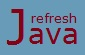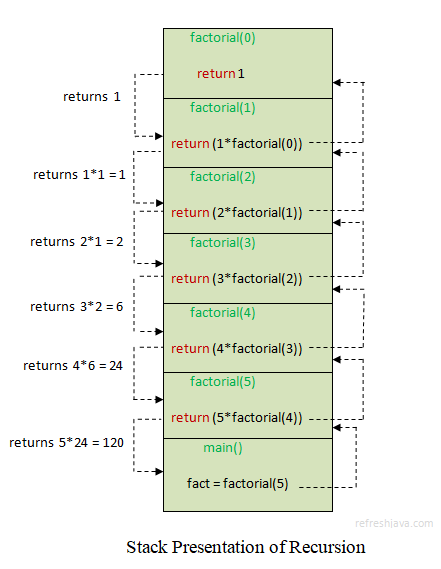# Method Chaining and Method Recursion in Java

This tutorial explains method chaining and method recursion concept. These are basically a mechanism related with method calling in java programs. Sometimes it is quite useful to use these concepts in our programs. We will see these concepts with program examples in this tutorial.

## Method Chaining in Java

Method chaining is a technique of calling multiple methods using dot(.) operator in a single statement. As the name itself suggests, it's a chain of method calls in a single statement. For example you might have already seen or used code like below :

```   StringBuilder str = `new` StringBuilder(`"java"`);
str.insert(0,`"refresh "`).append(`"tutorial "`).append(`"online"`); ` // Method chaining`
```

As you can see we are calling a series of `StringBuilder` method in a single statement. This is called method chaining. Each method except the last method in a method chaining syntax must return an object to allow the calls to be chained together, since you need an object to call a method.

Method chaining is possible on these method because each of these methods returns an object, which is then used to call the next method. For example the definition of `append` method of `StringBuilder` class is like below :

```    @Override
`public` StringBuilder append(String str) {
`super`.append(str);
`return this`;
}
```

The method is returning `this`, that is why we are able to invoke it multiple time in same line. In java `this` is a reference to the same object on which the method is being called. We will see the details of `this` keyword in later tutorial.

The program below demonstrates method chaining using the methods of `String` and `StringBuffer` classes. Methods like `insert, append, substring` etc allows us to use the technique of method chaining.

### Java program of method chaining using String and StringBuilder class methods

``` `class` MethodChainingProgram {
`public static void` main(String args[]) {
StringBuilder sb = `new` StringBuilder(`"Java"`);
`// Below line uses method chaining technique`
sb.insert(0,`"Learn "`).append(`" on refresh java"`).append(`" tutorial"`);
System.out.println(sb);
`// Below line also uses method chaining technique`
String str = sb.substring(14).replace(`" tutorial"`, `""`).toUpperCase();
System.out.println(str);
}
}
```

Output:

Learn Java on refresh java tutorial
REFRESH JAVA

Now let's create our own methods that can be used in method chaining approach. As mentioned above the method must return an object if it needs to be used in method chaining. The program below contains method `setName` and `setAge` that we can use in method chaining.

### Method chaining program in Java

``` `class` Person {
String name;
`int` age;

`// This method can be used in chaining`
`public` Person setName(String name) {
`this`.name = name;
`return this`;
}
`// This method can also be used in chaining`
`public` Person setAge(`int` age) {
`this`.age = age;
`return this`;
}
`public void` getPersonDetails() {
System.out.println(`"Person name :  "` + name + `", age : "` +age+`" year."`);
}
}

`class` MethodChainingDemo {
`public static void` main(String[] args) {
Person person= `new` Person();
`// Below line uses method chaining technique`
person.setName(`"Rahul"`).setAge(22).getPersonDetails();
}
}
```

Output:

Person name : Rahul, age : 22 year.

Using method chaining has couple of benefits like it improves the readability and reduces the amount of source code. But it also has some disadvantages as well like It's hard to debug if any of the method fails while execution. Exception handling also becomes tough, sometime it's confusing as well.

## Recursion in Java

Recursion is a process in which a method call itself from within it's body. The method that calls itself is known as recursive method. The code below shows an example of recursive method :

``` `int` calculate()
{
.....
calculate(); `//calling the same method`
.....
}
```

As you can see method `calculate` is calling itself, we can say `calculate` is a recursive method. Generally programmer uses some conditional/looping statements to call the same method. If we don't use any condition, the execution of method keep repeating itself again and again which results in an infinite recursion.

Recursion in computer science is a method of solving a problem. For example the program below calculates the factorial of a number using method recursion. Factorial of a number 5 is calculated as `5*4*3*2*1=120`.

### Factorial of a number using Recursion in Java

``` `class` FactorialProgram {

`static int` factorial(`int` n) {
`if`(n == 0)
`return` 1;
`else`
`return`(n * factorial(n-1));  `// Calling the same method`
}

`public static void` main(String args[]) {
`int` number=5;
`int` fact = factorial(number);
System.out.println(`"Factorial of number "`+number+`" is : "`+fact);
}
}
```

Output:

`Factorial of number 5 is : 120`

## How recursion works

As you know once a method is called, a new stack(memory) is allocated for method. This stack is used by method to store it's variables and performs it's operations and finally return the result to caller. In recursion every time the method is called, a new stack is allocated on the top of last stack. Once the last call happens, the method on top most stack evaluates it's result and returns the output to it's previous stack. The same process goes on until the method at first stack evaluates it's result and returns the result to it's caller.

For example in factorial program, once the main method calls the `findFactoria(5)`, it calls `findFactorial(4)` and it goes on until `findFactorial(0)` is called which returns 1 to `findFactorial(1)` which further returns it's result to `findFactorial(2)` and so on until `findFactorial(5)` is reached which evaluates the final result and returns to main method. The image below shows a typical representation of above factorial program.Programmer have to be careful while using method recursion, incorrect condition or logic may result in an infinite recursion. In an infinite recursion the method keeps calling itself again and again which means method call never ends. For example the program below results in an infinite recursion.

### Infinite recursion program in Java

``` `class` InfiniteRecursion {

`static void` printNumber(`int` num) {
`if`(num <= 10) {
System.out.println(num);
num = num-1;
printNumber(num);
}
}
`public static void` main(String args[]) {
printNumber(10);
}
}
```

Output:

```10
9
8
.
.
The condition statement that we have used in this program will always be `true`, as we are decreasing the number in each iteration. This will result in calling the method again and again. So you have to use the recursion carefully to avoid the infinite looping. If you change the conditions as `if`(num >= 1) the program will run successfully.